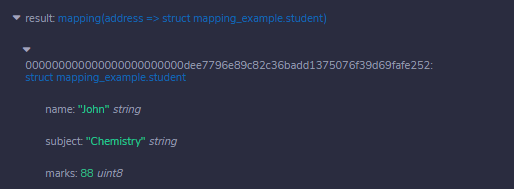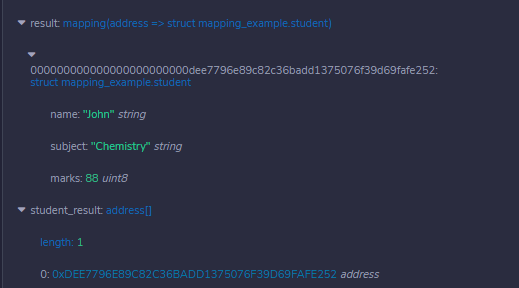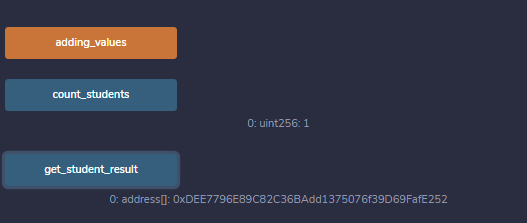# Solidity – Mappings

Mapping in Solidity acts like a hash table or dictionary in any other language. These are used to store the data in the form of key-value pairs, a key can be any of the built-in data types but reference types are not allowed while the value can be of any type. Mappings are mostly used to associate the unique Ethereum address with the associated value type.

Syntax:

```mapping(key => value) <access specifier> <name>;
```

### Creating a Mapping

Mapping is defined as any other variable type, which accepts a key type and a value type.

Example: In the below example, the contract mapping_example a structure is defined and mapping is created.

## Solidity

 `// Solidity program to  ` `// demonstrate mapping ` `pragma solidity ^0.4.18;  ` `  `  `// Defining contract  ` `contract` `mapping_example { ` `     `  `    ``//Defining structure ` `    ``struct` `student  ` `    ``{ ` `        ``// Declaring different  ` `        ``// structure elements ` `        ``string name; ` `        ``string subject; ` `        ``uint8 marks; ` `    ``} ` `     `  `    ``// Creating a mapping ` `    ``mapping ( ` `    ``address => student) result; ` `    ``address[] ``public` `student_result;     ` `}`

Output :As the mapping is created let’s try to add some values to the mapping for better understanding.

Example: In the below example, the contract mapping_example defines a structure, mapping is created and values are added to the mapping.

## Solidity

 `// Solidity program to  ` `// demonstrate adding  ` `// values to mapping ` `pragma solidity ^0.4.18;  ` ` `  `// Creating contract ` `contract` `mapping_example { ` `     `  `    ``//Defining structure  ` `    ``struct` `student { ` ` `  `        ``//Declaring different  ` `        ``// structure elements ` `        ``string name; ` `        ``string subject; ` `        ``uint8 marks; ` `    ``} ` ` `  `    ``// Creating mapping ` `    ``mapping ( ` `      ``address => student) result; ` `    ``address[] ``public` `student_result; ` `     `  `    ``// Function adding values to  ` `    ``// the mapping ` `    ``function adding_values() ``public` `{ ` `        ``var student  ` `          ``= result[0xDEE7796E89C82C36BAdd1375076f39D69FafE252]; ` ` `  `        ``student.name = ``"John"``; ` `        ``student.subject = ``"Chemistry"``; ` `        ``student.marks = 88; ` `        ``student_result.push( ` `          ``0xDEE7796E89C82C36BAdd1375076f39D69FafE252) -1; ` ` `  `    ``} ` `     `  `}`

Output :### Getting values

We have added values to the mapping, to retrieve the values we have to create a function that returns the values added to the mapping.

Example: In the below example, the contract mapping_example defines a structure, mapping is created, values are added to the mapping, and values are retrieved from the mapping.

## Solidity

 `// Solidity program to ` `// demonstrate retrieve ` `// values from the mapping ` ` `  `pragma solidity ^0.4.18;  ` `  `  `contract` `mapping_example { ` `     `  `    ``// Defining Structure ` `    ``struct` `student { ` ` `  `        ``// Declaring different data types ` `        ``string name; ` `        ``string subject; ` `        ``uint8 marks; ` `    ``} ` `     `  `    ``// Creating mapping ` `    ``mapping ( ` `      ``address => student) result; ` `    ``address[] student_result; ` `    `  `    ``// Function adding values to the mapping ` `    ``function adding_values() ``public` `{ ` `        ``var student  ` `          ``= result[0xDEE7796E89C82C36BAdd1375076f39D69FafE252]; ` ` `  `        ``student.name = ``"John"``; ` `        ``student.subject = ``"Chemistry"``; ` `        ``student.marks = 88; ` `        ``student_result.push( ` `          ``0xDEE7796E89C82C36BAdd1375076f39D69FafE252) -1; ` ` `  `    ``} ` `     `  `     ``// Function to retrieve  ` `     ``// values from a mapping ` `     ``function get_student_result( ` `     ``) view ``public` `returns (address[]) { ` `        ``return` `student_result; ` `    ``} ` `}`

Output :### Counting Mappings

Mappings can be counted so that we can know how many values are stored in mapping.

Example: In the below example, the contract mapping_example defines a structure, mapping is created, values are added to the mapping, and values are retrieved from the mapping.

## Solidity

 `// Solidity program to ` `// count number of  ` `// values in a mapping ` `pragma solidity ^0.4.18;  ` `  `  `contract` `mapping_example { ` `     `  `    ``// Defining structure ` `    ``struct` `student { ` ` `  `        ``// Declaring different ` `        ``// structure elements ` `        ``string name; ` `        ``string subject; ` `        ``uint8 marks; ` `    ``} ` `     `  `    ``// Creating mapping ` `    ``mapping (address => student) result; ` `    ``address[] student_result; ` `     `  `    ``//Function adding values to the mapping ` `    ``function adding_values() ``public` `{ ` `        ``var student ` `          ``= result[0xDEE7796E89C82C36BAdd1375076f39D69FafE252]; ` ` `  `        ``student.name = ``"John"``; ` `        ``student.subject = ``"Chemistry"``; ` `        ``student.marks = 88; ` `        ``student_result.push( ` `          ``0xDEE7796E89C82C36BAdd1375076f39D69FafE252) -1; ` ` `  `    ``} ` `     `  `     ``// Function to retrieve  ` `     ``// values from the mapping ` `     ``function get_student_result( ` `     ``) view ``public` `returns (address[]) { ` `        ``return` `student_result; ` `    ``} ` ` `  `    ``// Function to count number  ` `    ``// of values in a mapping ` `    ``function count_students( ` `    ``) view ``public` `returns (uint) { ` `        ``return` `student_result.length; ` `    ``} ` `}`

Output :My Personal Notes arrow_drop_upCheck out this Author's contributed articles.

If you like GeeksforGeeks and would like to contribute, you can also write an article using contribute.geeksforgeeks.org or mail your article to contribute@geeksforgeeks.org. See your article appearing on the GeeksforGeeks main page and help other Geeks.

Please Improve this article if you find anything incorrect by clicking on the "Improve Article" button below.

Article Tags :

Be the First to upvote.

Please write to us at contribute@geeksforgeeks.org to report any issue with the above content.